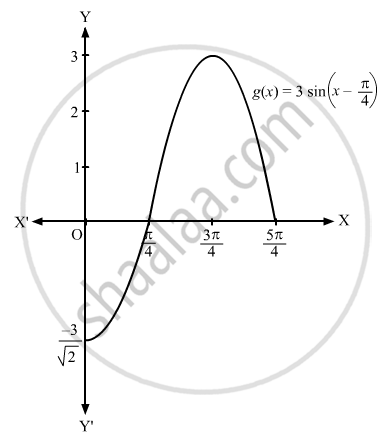Advertisement Remove all ads

# Sketch the Graph of the Following Function: F(X) = 2 Sin πX, 0 ≤ X ≤ 2 - Mathematics

Short Note

Sketch the graph of the following function:

$g\left( x \right) = 3 \sin \left( x - \frac{\pi}{4} \right), 0 \leq x \leq \frac{5\pi}{4}$

Advertisement Remove all ads

#### Solution

$g\left( x \right) = 3 \sin \left( x - \frac{\pi}{4} \right), 0 \leq x \leq \frac{5\pi}{4}$
 x $\frac{\pi}{4}$ $\frac{5\pi}{4}$ $g\left( x \right) = 3 \sin \left( x - \frac{\pi}{4} \right)$ 0 0Concept: Graphs of Trigonometric Functions
Is there an error in this question or solution?
Advertisement Remove all ads

#### APPEARS IN

RD Sharma Class 11 Mathematics Textbook
Chapter 6 Graphs of Trigonometric Functions
Exercise 6.1 | Q 1.2 | Page 5
Advertisement Remove all ads
Advertisement Remove all ads
Share
Notifications

View all notifications

Forgot password?